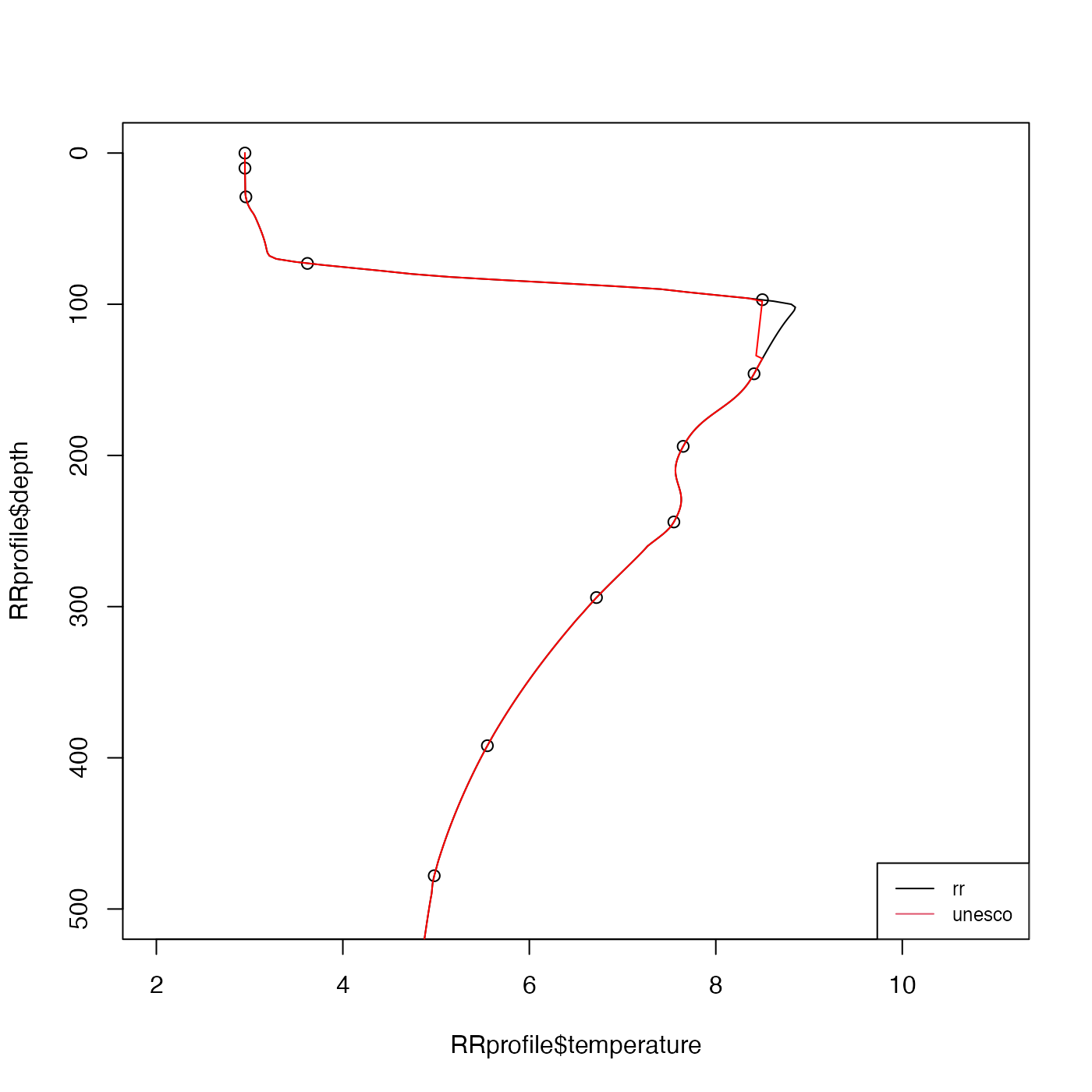Interpolate one-dimensional data using schemes that permit curvature but tends minimize extrema that are not well-indicated by the data.

oceApprox(x, y, xout, method = c("rr", "unesco"))

## Arguments

x

the independent variable (z or p, usually).

y

the dependent variable.

xout

the values of the independent variable at which interpolation is to be done.

method

method to use. See “Details”.

## Value

A vector of interpolated values, corresponding to the xout

values and equal in number.

## Details

Setting method="rr" yields the weighted-parabola algorithm of Reiniger and Ross (1968). For procedure is as follows. First, the interpolant for any xout value that is outside the range of x is set to NA. Next, linear interpolation is used for any xout value that has only one smaller neighboring x value, or one larger neighboring value. For all other values of xout, the 4 neighboring points x are sought, two smaller and two larger. Then two parabolas are determined, one from the two smaller points plus the nearest larger point, and the other from the nearest smaller point and the two larger points. A weighted sum of these two parabolas provides the interpolated value. Note that, in the notation of Reiniger and Ross (1968), this algorithm uses m=2 and n=1. (A future version of this routine might provide the ability to modify these values.)

Setting method="unesco" yields the method that is used by the U.S. National Oceanographic Data Center. It is described in pages 48-50 of reference 2; reference 3 presumably contains the same information but it is not as easily accessible. The method works as follows.

• If there are data above 5m depth, then the surface value is taken to equal to the shallowest recorded value.

• Distance bounds are put on the four neighboring points, and the Reiniger-Ross method is used for interpolated points with sufficiently four close neighbors. The bounds are described in table 15 of reference 2 only for so-called standard depths; in the present instance they are transformed to the following rules. Inner neighbors must be within 5m for data above 10m, 50m above 250m 100m above 900m, 200m above 2000m, or within 1000m otherwise. Outer neighbors must be within 200m above 500m, 400m above 1300m, or 1000m otherwise. If two or more points meet these criteria, Lagrangian interpolation is used. If not, NA is used as the interpolant.

After these rules are applied, the interpolated value is compared with the values immediately above and below it, and if it is outside the range, simple linear interpolation is used.

Dan Kelley

## Examples

library(oce)
if (require(ocedata)) {
data(RRprofile)
zz <- seq(0, 2000, 2)
plot(RRprofile$temperature, RRprofile$depth, ylim=c(500, 0), xlim=c(2, 11))
# Contrast two methods
a1 <- oce.approx(RRprofile$depth, RRprofile$temperature, zz, "rr")
a2 <- oce.approx(RRprofile$depth, RRprofile$temperature, zz, "unesco")
lines(a1, zz)
lines(a2, zz, col='red')
legend("bottomright",lwd=1,col=1:2, legend=c("rr","unesco"),cex=3/4)
}